# Graphing results from LiBiNorm

There are two options for producing graphical representations of the normalisation process using the three files that are produced using the -u <fileroot> option:

1. An R script is supplied that can produce graphs
2. An excel spreadsheet that functions on both Windows and a Mac is supplied that can produce graphs

### Producing graphs using the R script

The R script can be downloaded from here. It can be run using "Rscript LiBiNormPlot.R <fileroot>". It produces the following four graphs as png files alongside the text files from which they are derived. Note that the bias plot currently uses a different convention to that of figure 4A of the accompanying Cell Systems paper. A straight line through the origin in the paper would translate to a horizontal line at y = 1 in the graph produced by LiBiNorm.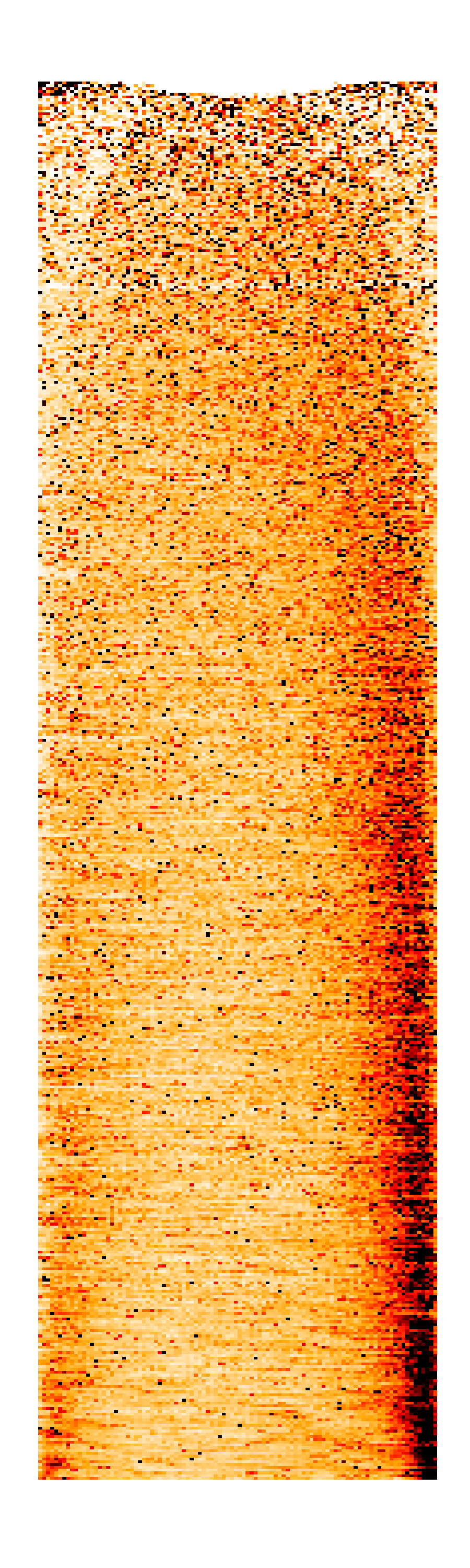Global distribution of sequencing reads. Detected transcripts are aligned at 5’ and 3’ ends and ordered by length, shortest on top. Read density along RNAs is indicated by colour intensity.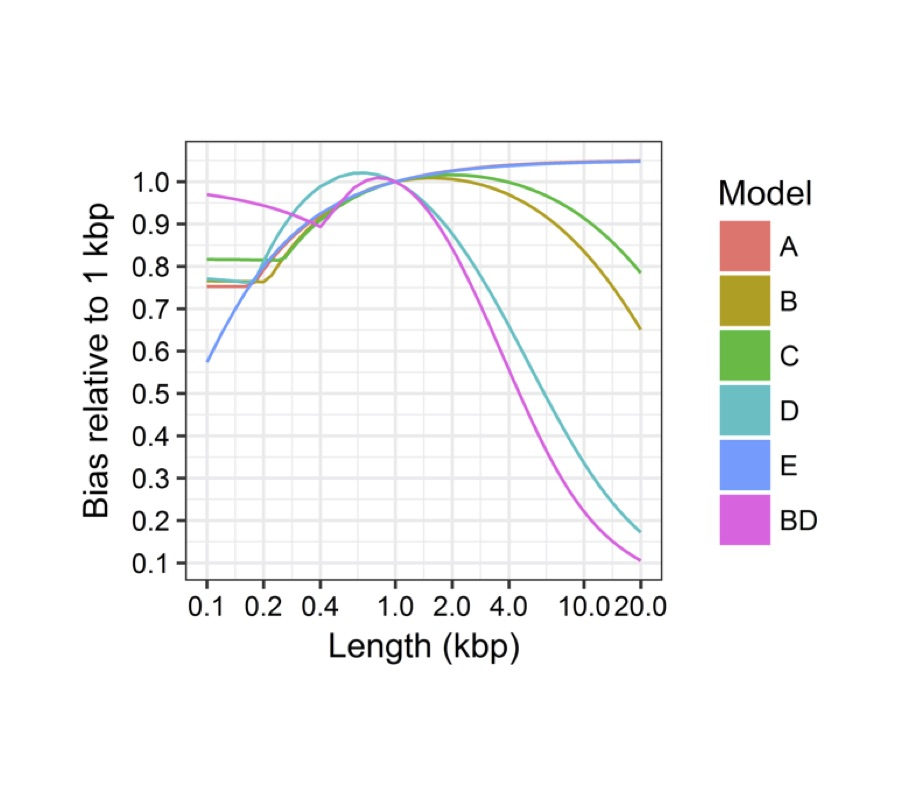Estimated global bias The prediced biases relative to a linear length model are plotted as a function of length for all fitted 6 models.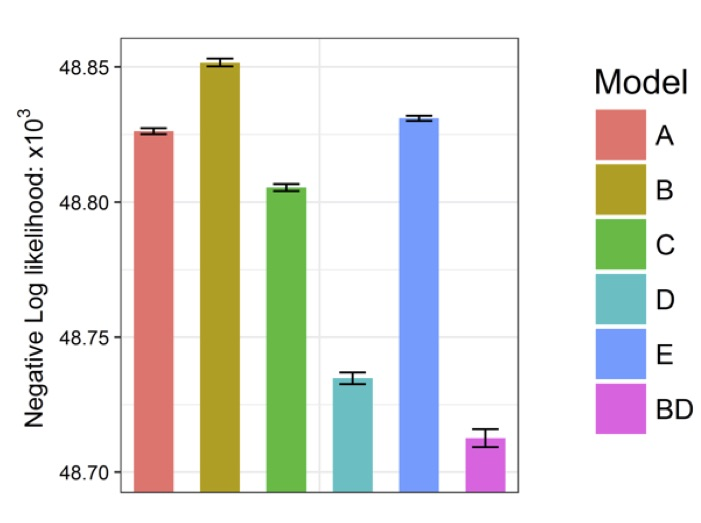Goodness-of-fit comparison (Negative) log likelihoods are shown for each model (the lower the better).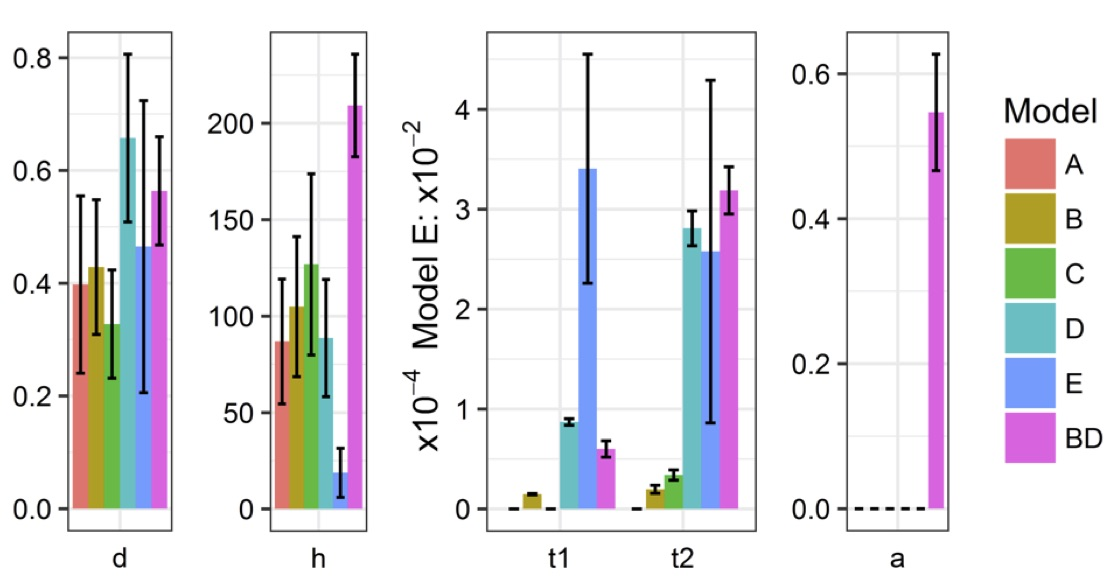Parameter estimates Interpretation: d + 1 ratio of fragmentation efficiencies inside strands vs close to ends h distance (bases) from ends over which fragmentation efficiency is reduced 1/t1 average synthesis length (bases) of reverse transcription (processivity) 1/t2 average synthesis length (bases) of second-strand synthesis (processivity) a fraction of PCR-selected full-length strands for SMART protocols
##### Running LiBiNormPlot.R

LiBiNormPlot.R requires four standard R packages in order to run: ggplot2, reshape2, gridExtra and scales. These may already be installed as part of your R installation. If they are not present, then LiBiNormPlot.R will generate an error message to that effect. The packages should be installed from within R (for example as described here). R may indicate that it does not have write access to the directory where packages are installed. If so, then one option is to set the environment variable R_LIBS to point to a directory where the packages can be installed, e.g. by including a line such as the following in a linux .profile file.

`export R_LIBS=/home/<username>/.local/lib/R/3.3 `

The plots are produced as lossless 300 ppi png bit maps. The ppi value is set at the start of the script and can be adjusted to change the resolution.

## Viewing results using an Excel spreadsheet

The results files can be viewed and plotted using this spreadsheet which works on both mac and Windows, using the browse button to locate the <filename>_results.txt file. The data can be reloaded using the Load button. Plots are available showing:

• The (negative) log likelihood for each model
• The predicted bias relative to a linear model for RNA from 200 to 20000 bp in length for each model
• The parameter estimates, and an indication of their uncertainty, for each model.

In addition the "Expression" tab shows the detailed counts, etc for each gene. The following graphs are available: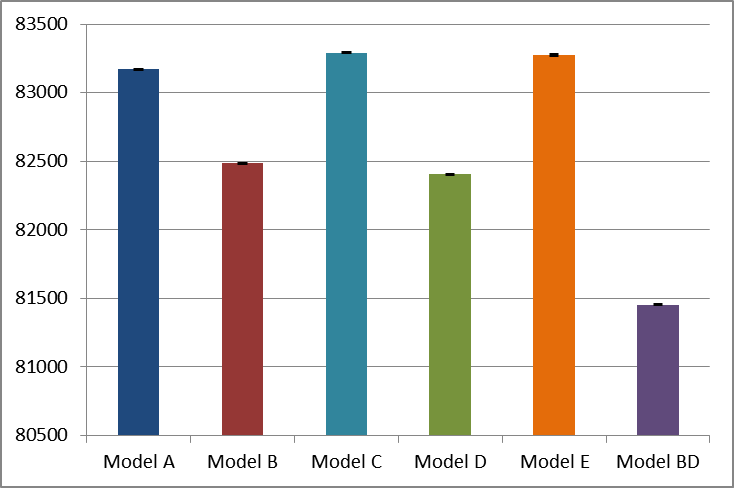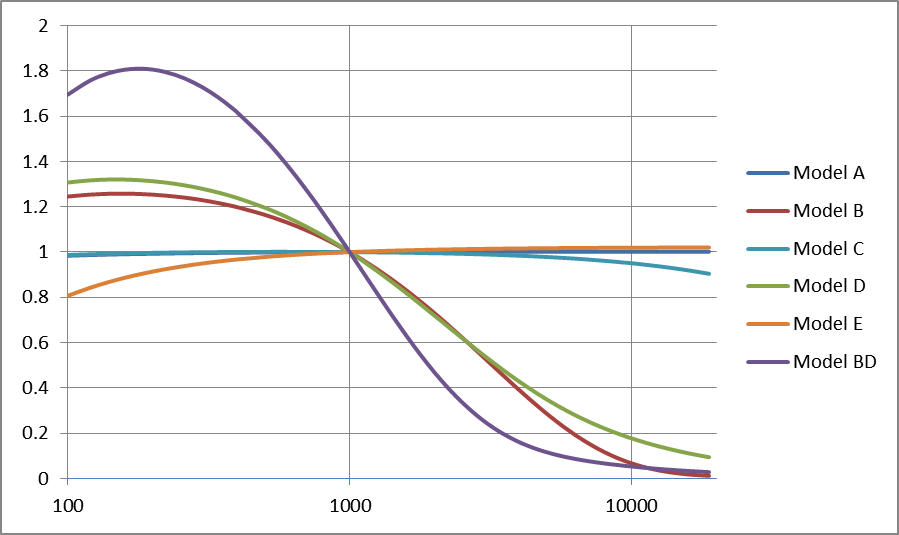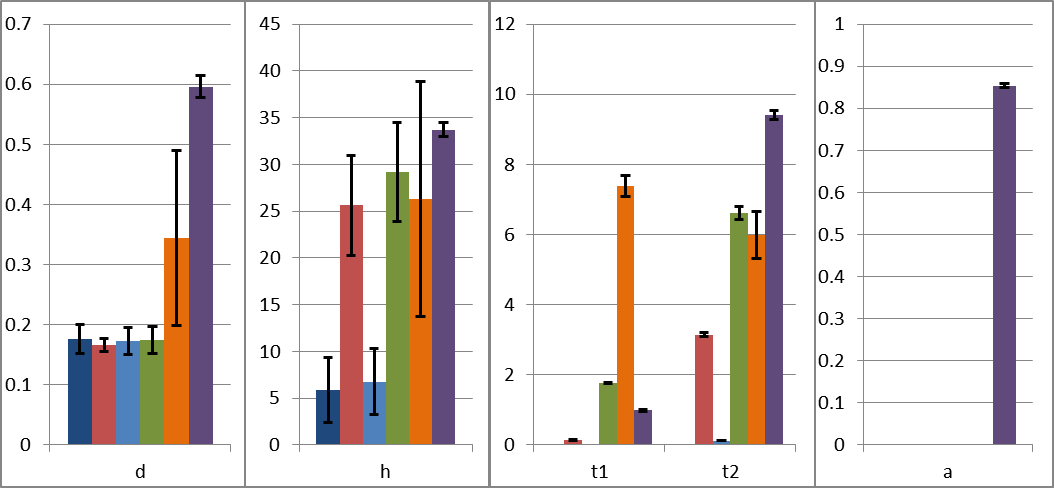The figures, their labels, and their interpretation are identical to the R figures above.

This approach allows the graphs to be copied and pasted as windows metafiles (i.e. vector graphics) into a word document which results in a smaller document size whilst retaining graphics quality. It can also be used as a starting point for generating vector graphics files for papers.